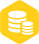# Option的基础用法

### 描述

Option 是 Rust 语言中的一个枚举类型，它表示一个值可能存在，也可能不存在的情况。Option 可以理解为一个容器，它可能装有一个值，也可能为空。在 Rust 中，Option 可以用来解决很多问题，比如判断一个值是否为空，避免空指针引用等。

Option 的定义如下：

``````enum Option< T > {
Some(T),
None,
}
``````

## 基础用法

### 创建 Option

Rust 中可以通过 `Some(value)` 创建一个包含值的 Option，也可以通过 `None` 创建一个空的 Option。

``````let some_value: Option< i32 > = Some(5);
let none_value: Option< i32 > = None;
``````

### 解构 Option

Option 中的值可以通过模式匹配的方式进行解构。例如，可以通过 `match` 表达式来判断 Option 中是否存在值，并进行相应的处理。

``````let some_value: Option< i32 > = Some(5);

match some_value {
Some(value) = > println!("The value is {}", value),
None = > println!("There is no value"),
}
``````

### 使用 unwrap

``````let some_value: Option< i32 > = Some(5);

let value = some_value.unwrap();
println!("The value is {}", value);
``````

### 使用 is_some 和 is_none

``````let some_value: Option< i32 > = Some(5);
let none_value: Option< i32 > = None;

println!("some_value is {}", some_value.is_some());
println!("none_value is {}", none_value.is_none());
``````

### 使用 map

``````let some_value: Option< i32 > = Some(5);

let new_value = some_value.map(|value| value * 2);
println!("The new value is {:?}", new_value);
``````

### 使用 and_then

``````let some_value: Option< i32 > = Some(5);

let new_value = some_value.and_then(|value| Some(value * 2));
println!("The new value is {:?}", new_value);
``````

## 进阶用法

### 使用 match 和 if let

``````let some_value: Option< i32 > = Some(5);

if let Some(value) = some_value {
println!("The value is {}", value);
} else {
println!("There is no value");
}
``````

### 使用 or 和 or_else

``````let some_value: Option< i32 > = Some(5);
let none_value: Option< i32 > = None;

let new_value = some_value.or(Some(10));
println!("The new value is {:?}", new_value);

let new_value = none_value.or(Some(10));
println!("The new value is {:?}", new_value);

let new_value = none_value.or_else(|| Some(10));
println!("The new value is {:?}", new_value);
``````

### 使用 filter

``````let some_value: Option< i32 > = Some(5);

let new_value = some_value.filter(|value| *value > 3);
println!("The new value is {:?}", new_value);

let new_value = some_value.filter(|value| *value > 10);
println!("The new value is {:?}", new_value);
``````

### 使用 take

``````let mut some_value: Option< i32 > = Some(5);

let value = some_value.take();
println!("The value is {:?}", value);
println!("The new Option is {:?}", some_value);
``````

## 实践经验

### 避免空指针引用

``````let mut some_value: Option< i32 > = Some(5);

let value = some_value.take();
if let Some(value) = value {
println!("The value is {}", value);
} else {
println!("There is no value");
}
``````

### 使用 Option 作为函数返回值

``````fn get_string() - > Option< String > {
let s = String::from("hello");
if s.is_empty() {
None
} else {
Some(s)
}
}
``````

### 使用 Option 作为结构体字段

``````struct Person {
name: Option< String >,
age: i32,
}

let person = Person {
name: Some(String::from("Tom")),
age: 18,
};
``````

### 使用 Option 和 Result 结合使用

``````fn get_string() - > Result< String, String > {
let s = String::from("hello");
if s.is_empty() {
Err(String::from("String is empty"))
} else {
Ok(s)
}
}

let result = get_string();
match result {
Ok(value) = > println!("The value is {}", value),
Err(value) = > println!("Error: {}", value),
}
``````

### 使用 Option 和 unwrap_or

``````let some_value: Option< i32 > = Some(5);
let none_value: Option< i32 > = None;

let new_value = some_value.unwrap_or(10);
println!("The new value is {}", new_value);

let new_value = none_value.unwrap_or(10);
println!("The new value is {}", new_value);
``````

### 使用 Option 和 Result 结合使用

``````fn get_string() - > Result< String, String > {
let s = String::from("hello");
if s.is_empty() {
Err(String::from("String is empty"))
} else {
Ok(s)
}
}

let result = get_string();
match result {
Ok(value) = > println!("The value is {}", value),
Err(value) = > println!("Error: {}", value),
}
``````

## 总结

Option 是 Rust 语言中一个非常重要的类型，它可以用来处理值可能存在或不存在的情况。在 Rust 中，Option 可以通过模式匹配、unwrap、is_some、is_none、map、and_then、or、or_else、filter、take 等方法来进行操作。使用 Option 可以避免空指针引用，保证程序的稳定性和安全性。同时，Option 和 Result 可以结合使用来处理错误和异常情况，提高程序的健壮性和可靠性。×
20